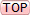nd order HIGH pass filter design TRANSFER FUNCTION $$H(s)=\dfrac{s^2}{s^2+2\zeta\omega_ns+\omega_n^2}$$   To find the "relevant" poles, equate the denominator to zero and solve for s: -   $$s = \dfrac{-2\zeta\omega_n\pm\sqrt{4\zeta^2\omega_n^2-4\omega_n^2}}{2} = -\zeta\omega_n\pm\sqrt{\zeta^2\omega_n^2-\omega_n^2}$$   $$s=\omega_n(-\zeta\pm\sqrt{\zeta^2-1})$$   Peaking frequency and amplitude$$H(j\omega)=\dfrac{1}{1-\dfrac{\omega_n^2}{\omega^2}-\dfrac{j2\zeta\omega_n}{\omega}}$$  The difference between high pass and low pass is that $$\omega_n$$ and $$\omega$$ have swapped places and the imaginary part is now negative. $$|H(j\omega)|=\dfrac{1}{\sqrt{(1-\dfrac{\omega_n^2}{\omega^2})^2+\dfrac{4\zeta^2\omega_n^2}{\omega^2}}}$$ Differentiate the denominator and equate to zero to find the minima: - $$0 = 4\omega_n^2 - 8\zeta^2\omega_n^2 - 4\dfrac{\omega_n^4}{\omega^2}$$ $$\omega^2 = \dfrac{4\omega_n^4}{4\omega_n^2 - 8\zeta^2\omega_n^2} = \dfrac{\omega_n^2}{1 - 2\zeta^2}$$     hence, $$\omega = \omega_n\sqrt{\dfrac{1}{1-2\zeta^2}}$$   Note the similarity with the low pass filter's peaking frequency: $$\omega = \omega_n\sqrt{1-2\zeta^2}$$   To find the peak magnitude, replace the peaking frequency in the formula for $$|H(j\omega)|$$: -  $$|H(j\omega)|=\dfrac{1}{\sqrt{(1-1+2\zeta^2)^2+4\zeta^2(1-2\zeta^2)}} = \dfrac{1}{\sqrt{4\zeta^4 + 4\zeta^2 - 8\zeta^4}}$$  $$=\dfrac{1}{2\zeta\sqrt{1-\zeta^2}}$$    i.e. exactly the same as for the low-pass filter example.

Quality factor, amplitude and phase at $$\omega_n$$$$H(j\omega)=\dfrac{1} {1-\dfrac{\omega^2}{\omega_n^2}-\dfrac{j2\zeta\omega}{\omega_n}}$$    and if $$\omega = \omega_n$$, then  $$H(j\omega)=\dfrac{1}{1-1-j2\zeta} = +jQ$$  $$=Q\angle{+90}$$

still under construction

Ω σ μ τ ω β δ η θ λ π ζ ∞ ° √ Δ ∂ ∫ Ʃ ± ≈ ≠ µ Ω ± ÷ Θ « » ≦ ≧ Σ Φ ε • θ λ ρ ω κ ² ³ ½ ¼ ¾ ° ∞ ∫≈ ≠ ≡ Ξ ∑ π Π © ®